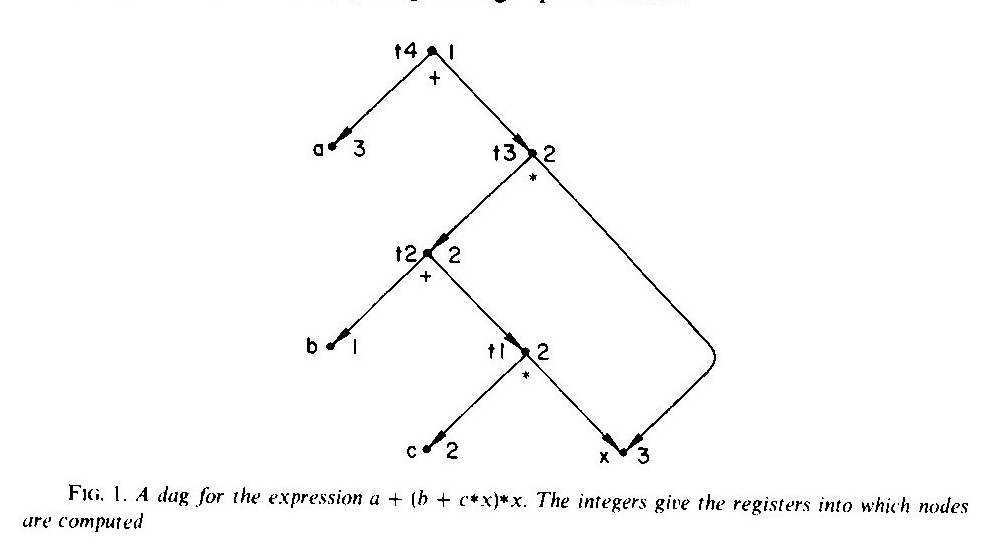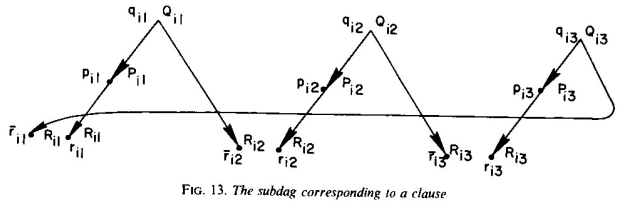# Tag Archives: PO2

## Feasible Register Assignment

On to a new section!  This section is on “Program Optimization”, and is the last “real” section- after this, there is a section of “Miscellaneous” problems and a section on “open” problems.  We’re heading towards the end!

PO1 is Register Sufficiency.  We’ll pick up with the next problem.

The problem: Feasible Register Assignment.  This is problem PO2 in the Appendix.

The description: Given a directed acyclic graph V = (G,A), a positive integer K, and a register assignment for each vertex (each vertex maps to one of K registers), can we create a computation for G (In the same sense as we did in Register Sufficiency) that also has the requirement that at each stage of the computation, we never have two vertices simultaneously assigned to the same register?

Thinking about this in terms of the “stones game” from the Register Sufficiency problem, we are saying that each vertex wants to use a specific stone, and so not only can we only use K stones (at most) at a time, we also can’t use the same stone for two different vertices that request that stone at the same time.

In the paper that has the reduction for this problem (and Register Sufficiency), Sethi makes the point that this means that we should keep a value in its register until all of its direct ancestors are computed.  So we are really asking if we can create a sequence of vertices where:

1. For all vertices u,v, and w in the sequence, if u is a direct descendant of w, and v appears between u and w, then u and v have different register allocations.
2. For all vertices u and v in the sequence, if u appears before v in the sequence, v is not a descendant of u

Example: Here is the example graph from Sethi’s paper that we used before:Suppose we had the following allocation of registers to vertices (this comes from p. 245 of the paper):

 node c x t1 b t2 t3 a t4 register 2 3 2 1 2 2 3 1

This allocation works if we use the sequence given in the table (c, then x, then t1, and so on).  We can see property 1 satisfied, for example, between t3 and x.  x is a direct descendant of t3. and so every vertex between x and t3 in the sequence cannot use x’s allocated register 3.  The second property is also true- we never have an ancestor vertex in the graph appear in the sequence before its descendant.

Here is a register allocation and sequence that will not work:

 node c x b a t1 t2 t3 t4 register 2 3 1 3 2 2 2 1

The problem here is again between t3 and x.  In this sequence, node a also uses register 3 in between, which violates property 1.

In programming terms, what is happening is that the second sequence copies the value of a into register 3, destroying the value of x that was already in there.  But the graph says we needed x to compute t3, and so by the time we got to the part of the sequence where we computed t3, we no longer had x’s value in a register.

Reduction: Sethi goes from 3SAT.  Our 3SAT instance will have n variables and m clauses.

The basic idea is that we will construct a graph with:

• One leaf vertex for the positive and negative versions of each literal (nodes sk and sk for variable k).  These vertices will both be allocated to register “Sk
• For each clause, construct the following subgraph:The lowercase letters are the vertex names.  The capital letters are the register assignments of the vertices (so, the q vertices map to registers “Qi1” through “Qi3“, and so on.  Note that both the positive and negative rij vertices both map to the same register “Rij“.
• Since r vertices represent literals in clauses, connect from them to the s vertices that represent the variables represented by the literal.  In other words, If the literal rij (literal j of clause i) is positive and represents variable xk, we connect an edge from rij to sk and from rij to sk.  If the literal is negative, we connect from rij to sk and from sk to rij

Suppose the formula is satisfiable.  Then if variable xk is true in the satisfying assignment, we add the vertices sk, all of its direct ancestors, and then sk to the sequence.  (In order from k=1 to n).  If xk is false, we add sk, then all of its ancestors, then sk.

We know that all of these ancestors are “r” vertices in clauses that have the variable and  that those “r” vertices only have 1 descendant (the s vertex corresponding to the setting of the variable.)  We also know that for all i and j, rij and rij have different children (they’ll be different parities of the corresponding s vertices).  This means we thus far have only added one vertex out of each rij and rij. Since each pair shared a register allocation (and were the only vertices sharing that register allocation), we’re ok so far.

If we have added the “positive” rij vertex to the sequence, next we will add the parent pij to the sequence.  This will “release” the need for the register allocation for rij, allowing us to add rij to the sequence.

After doing that for all rij, we need to handle the situation where we added the “negative” rij to the sequence, but not the “positive” version yet.  It turns out that in each clause, since the clause is satisfiable, we must have set its rij in the sequence already.  Let’s pretend that the literal that is true is literal 1.  So we know we have ri1 (and thus pi1) in the sequence, and since we have all “negated” r vertices, we must already also have ri2 in the sequence.  This lets us allocate the vertex qi1, which lets us release the register allocation of Ri2 that was held by ri2, letting us add ri2 to the sequence, and then we can follow that process around the clause widget.  This will then let us add all Q vertices to the sequence, giving us a legal ordering of the vertices.

The other direction is a little more convoluted.  First, he shows that if we have a pair of “parent” vertices (u1 and u2) with the same register allocation, and those  parents each have child vertices (v1 and v2) with the same register allocation, then u1 comes before u2 in a legal sequence iff v1 comes before v2 in that sequence.  Since parents have to come after children n the sequence, the only for that not to happen is if we had a sequence v2, v1, u1, u2.  But that sequence forces us to visit both v1 and v2 while they are both holding the same register, which can’t happen.

Next, we look at a sequence for the graph we build from a satisfiability instance.  He shows that if we have a legal sequence there must be, for all i, some rij that appears before the corresponding rij.  If that wasn’t the case, we’d have no legal way to get to the Q vertices, since the rij will hold its register allocation the whole time, meaning we can’t ever put the positive rij in the sequence.

This vertex that has its rij first corresponds to a literal being set to true in a clause and gives us a way to satisfy the entire formula.

Difficulty: 8.  This is a pretty tricky construction, even if it is similar to other SAT reductions we’ve seen.  But the need to add the extra p vertex on the positive (but not negative) side, and the Q vertex having as descendants rij and ri(j+1) make this hard to follow.